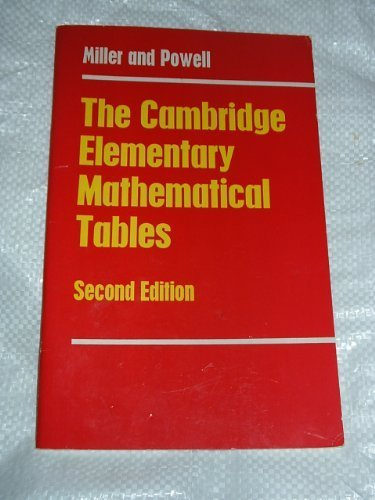# The Cambridge Elementary Mathematical Tables by J.C.P. Miller

In Stock
\$16.99
+10% GST
Second edition, first published in 1965. Compiled and arranged for the Cambridge Local Examinations Syndicate.
Only 2 left

## The Cambridge Elementary Mathematical Tables Summary

### The Cambridge Elementary Mathematical Tables by J.C.P. Miller

Second edition, first published in 1965. Compiled and arranged for the Cambridge Local Examinations Syndicate.

### Why buy from World of BooksOur excellent value books literally don't cost the earthFree delivery in AustraliaEvery used book bought is one saved from landfill

Notes on the use of the tables; Logarithms; Antilogarithms; Logarithms of sines; Logarithms of cosines; Minutes to decimals of a degree; logarithms of tangents; Sines; Cosines; proportional parts for sixths; Tangents; Secants; Squares; Square roots; Reciprocals; Powers and factorials; Degrees, minutes and radians; Natural logarithms; Exponential, hyperbolic and circular functions; Binomial coefficients; Binomial distribution; t-distributions; Chi-square distributions; Correclation coefficients; Proportional parts for tenths; international system of units (SI); Physical constants.

GOR003187240
The Cambridge Elementary Mathematical Tables by J.C.P. Miller
J.C.P. Miller